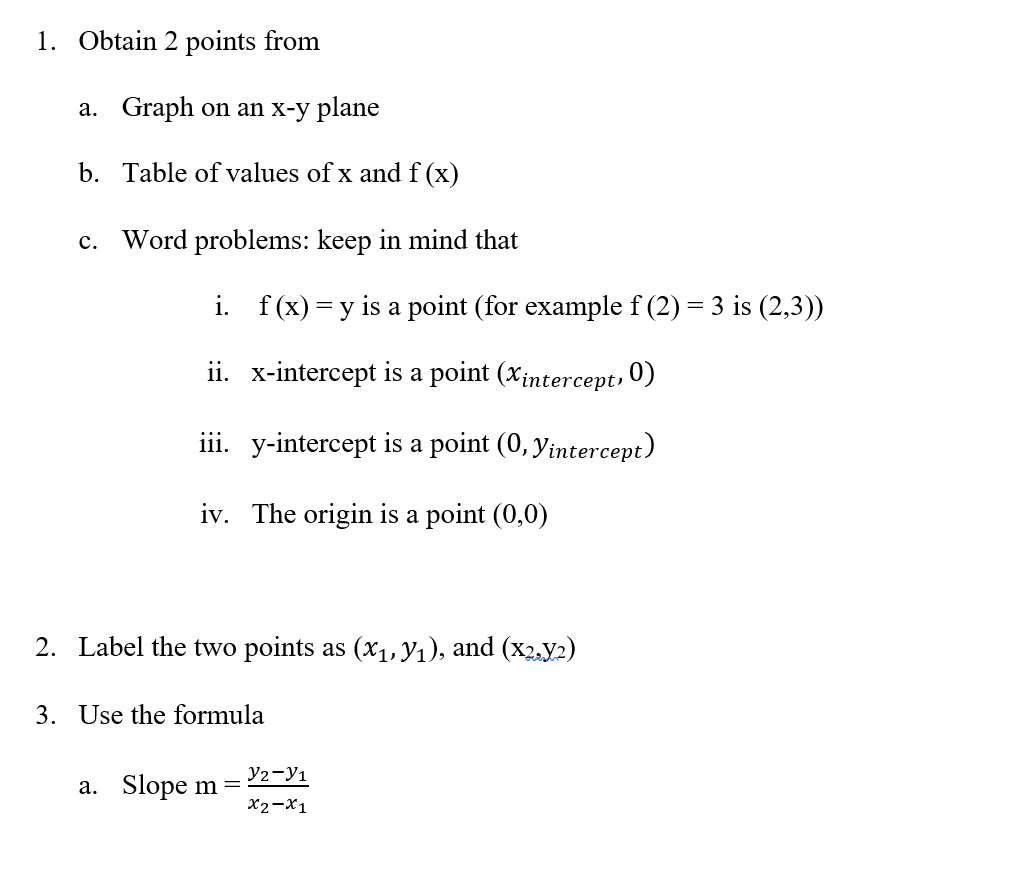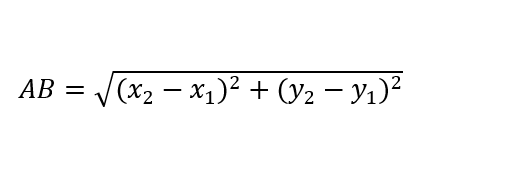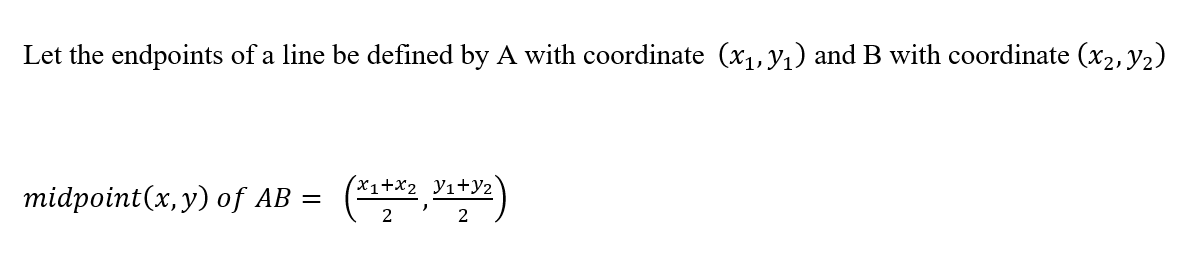Linear Functions and Equations review

What are the three forms of Linear equations?How to find slope when you are given two pointsHow to solve word problems with linear equations intercept form.How to write linear equationsHow to find the distance between two points on an x-y plane.How to find the midpoint of a line segment on an x-y plane.